# Abstract¶

## Implicit Matrix Factorization¶

The Implicit Matrix Factorization (IMF) algorithm as presented by Hu, Koren and Volinksy is a widely popular, effective method for recommending items to users. This approach was born from necessity: while explicit feedback as to our users' tastes - a questionnaire completed at user signup, for example - makes building a recommendation engine straightforward, we often have nothing more than implicit feedback data - view count, click count, time spent on page, for example - which serve instead as a proxy for these preferences. Crucially, the latter feedback is asymmetric: while a high view count might indicate positive preference for a given item, a low view count cannot be said to do the opposite. Perhaps, the user simply didn't know the item was there.

IMF begins with a ratings matrix $R$, where $R_{u, i}$ gives the implicit feedback value observed for user $u$ and item $i$. Next, it constructs two other matrices defined as follows:

$$p_{u, i} = \begin{cases} 1 & r_{u, i} \gt 0\\ 0 & r_{u, i} = 0 \end{cases}\\ c_{u, i} = 1 + \alpha r_{u, i}$$

$P$ gives a binary matrix indicating our belief in the existence of each user's preference for each item. $C$ gives our confidence in the existence of each user's preference for each item where, trivially, larger values of $r_{u, i}$ give us higher confidence that user $u$ indeed likes item $i$.

Next, IMF outlines its goal: let's embed each user and item into $\mathbb{R}^f$ such that their dot product approximates the former's true preference for the latter. Finally, and naming user vectors $x_u \in \mathbb{R}^f$ and item vectors $y_i \in \mathbb{R}^f$, we compute the argmin of the following objective:

$$\underset{x_{*}, y_{*}}{\arg\min}\sum\limits_{u, i}c_{u, i}\big(p_{u, i} - x_u^Ty_i\big)^2 + \lambda\bigg(\sum\limits_u\|x_u\|^2 + \sum\limits_i\|y_u\|^2\bigg)$$

Once sufficiently minimized, we can compute expected preferences $\hat{p}_{u, i} = x_u^Ty_i$ for unobserved $\text{(user, item)}$ pairs; recommendation then becomes:

1. For a given user $u$, compute predicted preferences $\hat{p}_{u, i} = x_u^Ty_i$ for all items $i$.
2. Sort the list in descending order.
3. Returning the top $n$ items.

## Shallow neural networks¶

IMF effectively gives a function $f: u, i \rightarrow \hat{p}_{u, i}$. As before, our goal is to minimize the function above, which we can now rewrite as:

$$\underset{x_{*}, y_{*}}{\arg\min}\sum\limits_{u, i}c_{u, i}\big(p_{u, i} - f(u, i)\big)^2 + \lambda\bigg(\sum\limits_u\|x_u\|^2 + \sum\limits_i\|y_u\|^2\bigg)$$

To approximate this function, I turn to our favorite universal function approximator: neural networks optimized with stochastic gradient descent.

This work is built around a toy web application I authored long ago: dotify. At present, dotify:

• Pulls data nightly from Spotify Charts. These data contain the number of streams for that day's top 200 songs for each of 55 countries.
• Computes an implicit matrix factorization nightly, giving vectors for both countries and songs.
• Allows the user to input a "country-arithmetic" expression, i.e. "I want music like Colombia x Turkey - Germany." It then performs this arithmetic with the chosen vectors and recommends songs to the composite.

In this work, I first fit and cross-validate an implicit matrix factorization model, establishing the three requisite parameters: $f$, the dimensionality of the latent vectors; $\alpha$, the scalar multiple used in computing $C$; $\lambda$ the regularization strength used in on our loss function.

### Network architectures¶

Next, I explore five different shallow neural network architectures in attempt to improve upon the observed results. These architectures are as follows:

1. A trivially "Siamese" network which first embeds each country and song index into $\mathbb{R}^f$ in parallel then computes a dot-product of the embeddings. This is roughly equivalent to what is being done by IMF.
2. Same as previous, but with a bias embedding for each set, in $\mathbb{R}$, added to the dot-product.
3. Same as #1, but concatenate the vectors instead. Then, stack 3 fully-connected layers with ReLU activations, batch normalization after each, and dropout after the first. Finally, add a 1-unit dense layer on the end, and add bias embeddings to the result. (NB: I wanted to add the bias embeddings to the respective $\mathbb{R}^f$ embeddings at the outset, but couldn't figure out how to do this in Keras.)
4. Same as #2, except feed in the song title text as well. This text is first tokenized, then padded to a maximum sequence length, then embedded into a fixed-length vector by an LSTM, then reduced to a single value by a dense layer with a ReLU activation. Finally, this scalar is concatenated to the scalar output that #2 would produce, and the result is fed into a final dense layer with a linear activation - i.e. a linear combination of the two.
5. Same as #4, except feed in the song artist index as well. This index is first embedded into a vector, then reduced to a scalar by a dense layer with a ReLU activation. Finally, this scalar is concatenated with the two scalars produced in the second-to-last layer of #4, then fed into a final dense layer with a linear activation. Like the previous, this is a linear combination of the three inputs.

# Results¶

While the various network architectures are intruiging for both the ease with which we can incorporate multiple, disparate inputs into our function, and the ability to then train this function end-to-end with respect to our main minimization objective, we find no reason to prefer them over the time-worn Implicit Matrix Factorization algorithm.

# Data preparation¶

To construct our ratings matrix, I take the sum of total streams for each $\text{(country, song)}$ pair. The data are limited to a given number of "top songs," defined as a song that appeared on Spotify Charts on a given date.

Because the values exist on wildly different orders of magnitude, I scale the results as follows:

$$\tilde{r}_{u, i} = \log{\bigg(\frac{1 + r_{u, i}}{\epsilon}\bigg)}$$

To start, let's build a ratings matrix for a small sample of the data.

In :
ratings_matrix = RatingsMatrix(n_top_songs=10000, eps=1e3)

Out:
song_id 5552 5553 5557 5558 5560 5562 5565 5582 5583 5585 ... 33062 33064 33065 33066 33067 33068 33069 33070 33071 33072
country_id
1 0.000000 0.0 0.0 0.000000 0.0 0.0 0.0 0.000000 0.0 0.0 ... 0.0 0.000000 0.000000 0.0 0.0 0.0 0.0 0.0 0.0 0.0
2 0.000000 0.0 0.0 0.000000 0.0 0.0 0.0 3.643437 0.0 0.0 ... 0.0 0.000000 0.000000 0.0 0.0 0.0 0.0 0.0 0.0 0.0
4 2.546472 0.0 0.0 3.597833 0.0 0.0 0.0 0.000000 0.0 0.0 ... 0.0 0.000000 0.000000 0.0 0.0 0.0 0.0 0.0 0.0 0.0
5 0.000000 0.0 0.0 3.175760 0.0 0.0 0.0 0.000000 0.0 0.0 ... 0.0 0.000000 0.000000 0.0 0.0 0.0 0.0 0.0 0.0 0.0
6 0.000000 0.0 0.0 0.000000 0.0 0.0 0.0 0.000000 0.0 0.0 ... 0.0 1.138153 0.961264 0.0 0.0 0.0 0.0 0.0 0.0 0.0

5 rows × 2110 columns

Next, let's get an idea of the sparsity of our data and how many songs each country has streamed.

In :
sparsity = (ratings_matrix.R_ui == 0).mean().mean()
non_zero_entries_percent = 100*np.round(1 - sparsity, 4)

print('Our ratings matrix has {}% non-zero entries.'.format(non_zero_entries_percent))

Our ratings matrix has 7.3999999999999995% non-zero entries.

In :
print('Our ratings matrix contains {} countries and {} unique songs.'.format(*ratings_matrix.R_ui.shape))

Our ratings matrix contains 55 countries and 2110 unique songs.

In :
plt.figure(figsize=(10, 6))

songs_rated_by_country = (ratings_matrix.R_ui > 0).sum(axis=1)
plt.hist(songs_rated_by_country)
plt.title('Distribution of # of Unique Songs Streamed per Country', fontsize=15)
plt.xlabel('Unique Songs Streamed', fontsize=12)
_ = plt.ylabel('# of Countries', fontsize=12)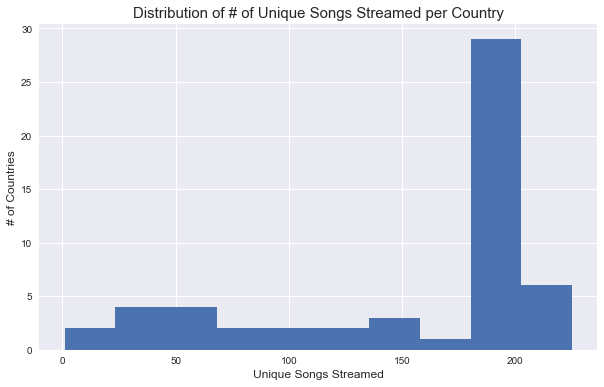## Construct training, validation sets¶

In reviewing literature on recommender engine evaluation, it seems common to create training and validation sets as follows:

1. Filter your ratings matrix for users (countries) that meet some criterion, i.e. they've streamed above a certain threshold of songs.
2. Select a random $x\%$ of their items (songs).
3. "Move" these items into a validation matrix; set them to 0 in the training matrix.

To construct this split, I first compute a sensible threshold for Step #1, then "move" a random 20% of songs from the training matrix to the validation matrix.

In :
print('The 15th percentile of songs rated by country is {}.'.format(
np.percentile(songs_rated_by_country, 15)
))

The 15th percentile of songs rated by country is 54.5.


Let's choose 50 songs as our cutoff, and move 20% of the songs in qualifying rows to a validation set.

NB: The actual ratings matrix is located at RatingsMatrix.R_ui. This reflects an API choice I made when first starting this project.

In :
def split_ratings_matrix_into_training_and_validation(ratings_matrix, eligibility_mask, fraction_to_drop=.2):
training_matrix = ratings_matrix
validation_matrix = deepcopy(training_matrix)
validation_matrix.R_ui = pd.DataFrame(0., index=training_matrix.R_ui.index, columns=training_matrix.R_ui.columns)

n_songs_to_drop = int( len(rated_songs)*fraction_to_drop )
songs_to_drop = set( random.sample(rated_songs, n_songs_to_drop) )

validation_matrix.R_ui.ix[country_id][songs_to_drop] = training_matrix.R_ui.ix[country_id][songs_to_drop]
training_matrix.R_ui.ix[country_id][songs_to_drop] = 0.

return training_matrix, validation_matrix

In :
more_than_50_ratings_mask = songs_rated_by_country > 50

training_matrix, validation_matrix = split_ratings_matrix_into_training_and_validation(
ratings_matrix=ratings_matrix,
)


# Evaluation¶

Evaluating recommender systems is an inexact science because there is no "right" answer. In production, this evaluation is often done via the A/B test of a proxy metric important to the business - revenue, for example. In training, the process is less clear. To this end, the authors of the IMF paper offer the following:

Evaluation of implicit-feedback recommender requires appropriate measures. In the traditional setting where a user is specifying a numeric score, there are clear metrics such as mean squared error to measure success in prediction. However with implicit models we have to take into account availability of the item, competition for the item with other items, and repeat feedback. For example, if we gather data on television viewing, it is unclear how to evaluate a show that has been watched more than once, or how to compare two shows that are on at the same time, and hence cannot both be watched by the user.

It is important to realize that we do not have a reliable feedback regarding which programs are unloved, as not watching a program can stem from multiple different reasons. In addition, we are currently unable to track user reactions to our recommendations. Thus, precision based metrics are not very appropriate, as they require knowing which programs are undesired to a user. However, watching a program is an indication of liking it, making recall-oriented measures applicable.

In solution, they propose evaluating the "expected percentile ranking" defined as follows:

$$\overline{\text{rank}} = \frac{\sum_{u, i}\tilde{r}_{u, i}^t\text{rank}_{u, i}}{\sum_{u, i}\tilde{r}_{u, i}^t}$$

Here, $\text{rank}_{u, i}$ gives the percentile-ranking of the predicted preference, i.e. if $\hat{p}_{u = 17, i = 34}$ is the largest of all predicted preferences, then $\text{rank}_{u = 17, i = 34} = 0\%$. Similarly, the smallest of the predicted preferences, i.e. the last on the list, equals $100\%$.

The following class accepts a training matrix, validation matrix and a matrix of predicted preferences. It then exposes the mean expected percentile ranking for both training and validation sets as properties on the instance.

In :
class ExpectedPercentileRankingsEvaluator:

def __init__(self, training_matrix, validation_matrix, eligibility_mask, predicted_preferences):
self.training_matrix = training_matrix
self.validation_matrix = validation_matrix
self.predicted_preferences = predicted_preferences
self._expected_percentile_rankings_train = []
self._expected_percentile_rankings_validation = []

def run(self):
self._evaluate_train()
self._evaluate_validation()

def _evaluate_train(self):
self._expected_percentile_rankings_train = self._evaluate(matrix=self.training_matrix)

def _evaluate_validation(self):
self._expected_percentile_rankings_validation = self._evaluate(matrix=self.validation_matrix)

def _evaluate(self, matrix):
expected_percentile_rankings = []
predictions = pd.DataFrame({
'predicted_preference': preferences.sort_values(ascending=False),
'rank': np.arange( len(preferences) ),
'percentile_rank': np.arange( len(preferences) ) / len(preferences)
})
ground_truth = matrix.R_ui.ix[country_id][ matrix.R_ui.ix[country_id] > 0 ]
numerator = (ground_truth * predictions['percentile_rank'][ground_truth.index]).sum()
denominator = ground_truth.sum()
expected_percentile_rankings.append( numerator / denominator )

return expected_percentile_rankings

@property
def mean_expected_percentile_rankings_train(self):
return np.mean(self._expected_percentile_rankings_train)

@property
def mean_expected_percentile_rankings_validation(self):
return np.mean(self._expected_percentile_rankings_validation)


Next, we perform a basic grid search to find reasonable values for $\alpha$ and $\lambda$.

In [ ]:
F = 30
grid_search_results = {}
result = namedtuple('Result', 'alpha lmbda')
alpha_values = [1e-1, 1e0, 1e1, 1e2]
lmbda_values = [1e-1, 1e0, 1e1, 1e2]

for alpha in alpha_values:
for lmbda in lmbda_values:
implicit_mf = ImplicitMF(ratings_matrix=training_matrix, f=F, alpha=alpha, lmbda=lmbda)
implicit_mf.run()
predicted_preferences = implicit_mf.country_vectors.vectors.dot( implicit_mf.song_vectors.vectors.T )

evaluator = ExpectedPercentileRankingsEvaluator(
training_matrix=training_matrix,
validation_matrix=validation_matrix,
predicted_preferences=predicted_preferences
)
evaluator.run()
grid_search_results[result(alpha=alpha, lmbda=lmbda)] = {
'train': evaluator.mean_expected_percentile_rankings_train,
'validation': evaluator.mean_expected_percentile_rankings_validation
}
print(grid_search_results)


Let's visualize the results for clarity. I plot the opposite of the validation score such that the parameters corresponding to the darkest square are most favorable.

In :
grid_search_items = [(params, results) for params, results in grid_search_results.items()]
grid_search_items.sort(key=lambda tup: tup.alpha)

params, results = zip(*grid_search_items)
validation_results = np.array([result['validation'] for result in results]).reshape(4, 4)

plt.figure(figsize=(15, 10))
plt.xticks([])
plt.yticks([])
plt.pcolormesh(-validation_results, cmap='Reds')
plt.colorbar()
for alpha_index, alpha in enumerate(alpha_values):
for lbmda_index, lmbda in enumerate(lmbda_values):
plt.text(
x = lbmda_index + 0.5,
y = alpha_index + 0.5,
s = r'$\alpha$ = {}'.format(alpha) + '\n' + r'$\lambda$ = {}'.format(lmbda),
ha='center',
va='center',
size=15,
color='w'
)
plt.title('Grid Search Results', fontsize=15)
plt.xlabel(r'$\lambda$ Values', fontsize=14)
_ = plt.ylabel(r'$\alpha$ Values', fontsize=14)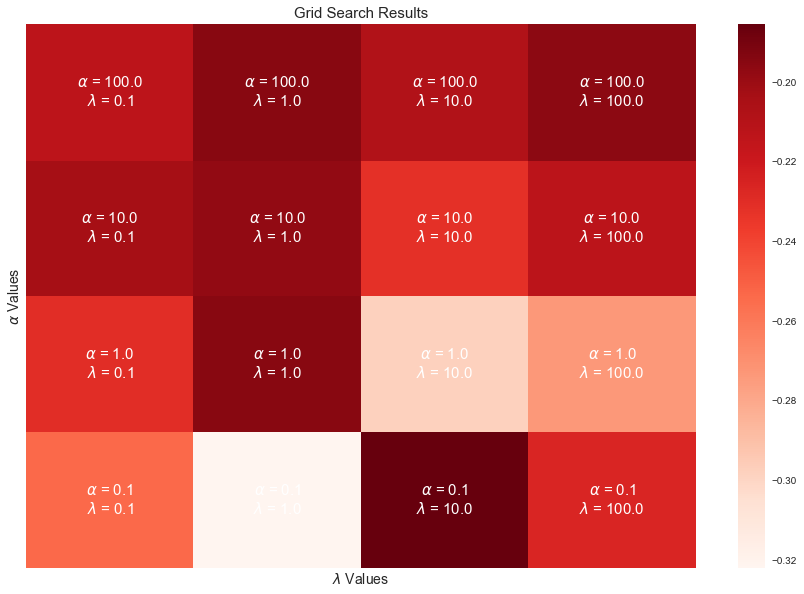In :
best_params = min(grid_search_results, key=lambda key: grid_search_results.get(key)['validation'])
print('The best parameters were found to be: {}.'.format(best_params))

The best parameters were found to be: Result(alpha=0.1, lmbda=10.0).


# Train a final model¶

Using these parameters, let's train a final implicit matrix factorization model using 1,000,000 top songs. Additionally, we'll choose $\epsilon = 1,000$ which seemed to work best in simple experimentation. This experimentation is not shown here.

In :
ratings_matrix = RatingsMatrix(n_top_songs=1000000, eps=1e3)

In :
print('Our ratings matrix contains {} countries and {} unique songs.'.format(*ratings_matrix.R_ui.shape))

Our ratings matrix contains 55 countries and 8663 unique songs.

In [ ]:
implicit_mf = ImplicitMF(ratings_matrix=ratings_matrix, f=F, alpha=best_params.alpha, lmbda=best_params.lmbda)
implicit_mf.run()


# Visualize¶

Let's plot the cosine similarities between all pairs of countries to confirm that things make sense. Intuitively, I'd think that countries in the following groups should be similar:

• United States, United Kingdom, Canada, Australia, New Zealand
• Latin American countries
• Sweden, Finland, Norway, Denmark

First let's replace the index of our vectors such that it contains the country names.

In :
country_vectors_df = implicit_mf.country_vectors.vectors.copy()

country_id_to_name = {countries_lookup[name]['id']: name for name in countries_lookup}
country_ids = country_vectors_df.index
country_names = pd.Index([country_id_to_name[c_id] for c_id in country_ids], name='country_name')
country_vectors_df.index = country_names


Out:
dim_0 dim_1 dim_2 dim_3 dim_4 dim_5 dim_6 dim_7 dim_8 dim_9 ... dim_20 dim_21 dim_22 dim_23 dim_24 dim_25 dim_26 dim_27 dim_28 dim_29
country_name
United States -1.276051 -1.058583 -0.622779 0.430231 0.306830 0.966786 0.190530 -0.549356 0.538788 -0.722162 ... 0.057478 0.500288 0.799253 0.383243 0.575051 0.247066 0.548018 -0.238643 0.114481 0.731294
United Kingdom 0.106521 0.346207 -0.276669 -0.003249 -1.294024 -0.090318 0.666301 -0.533962 0.281620 -0.268332 ... -0.803658 -0.498773 -0.041302 -0.705815 0.624482 -0.007293 1.335080 -0.717667 0.019507 -1.700016
Argentina -0.871966 0.678408 0.343354 -0.968614 -1.099471 0.014175 0.031833 -0.370821 -0.114270 -0.982766 ... 0.435942 -0.170878 -0.172491 -0.158596 0.445267 -0.949628 0.542433 -0.157643 0.765119 0.587947
Australia -1.129085 0.212391 0.136416 -0.665215 0.452084 -0.418566 -0.187979 0.807976 -0.693282 -0.723989 ... -0.961785 -0.064047 -0.161033 0.211444 0.180285 0.597487 -0.143220 0.336241 -0.251201 -1.057264
Austria -0.094485 0.870111 -0.328650 -0.594735 -0.429165 -0.955718 0.151173 0.250305 -0.302996 0.718965 ... -0.409435 -0.381884 -0.144373 0.340587 0.006711 0.870717 0.067101 -0.361675 0.742727 1.341254

5 rows × 30 columns

Then, we'll plot the cosine similarities.

In :
sns.set(style="white")

def plot_cosine_similarities(country_vectors_df):

similarities_df = pd.DataFrame(
data=cosine_similarity(country_vectors_df),
index=country_vectors_df.index,
columns=country_vectors_df.index
)

f, ax = plt.subplots(figsize=(21, 21))
cmap = sns.diverging_palette(220, 10, as_cmap=True)

sns.heatmap(
similarities_df,
cmap=cmap,
vmax=.5,
square=True,
xticklabels=True,
yticklabels=True,
linewidths=1,
cbar_kws={"shrink": .5},
ax=ax,
)
ax.set_title('Cosine Similarity Matrix', fontsize=20)

In :
plot_cosine_similarities(country_vectors_df)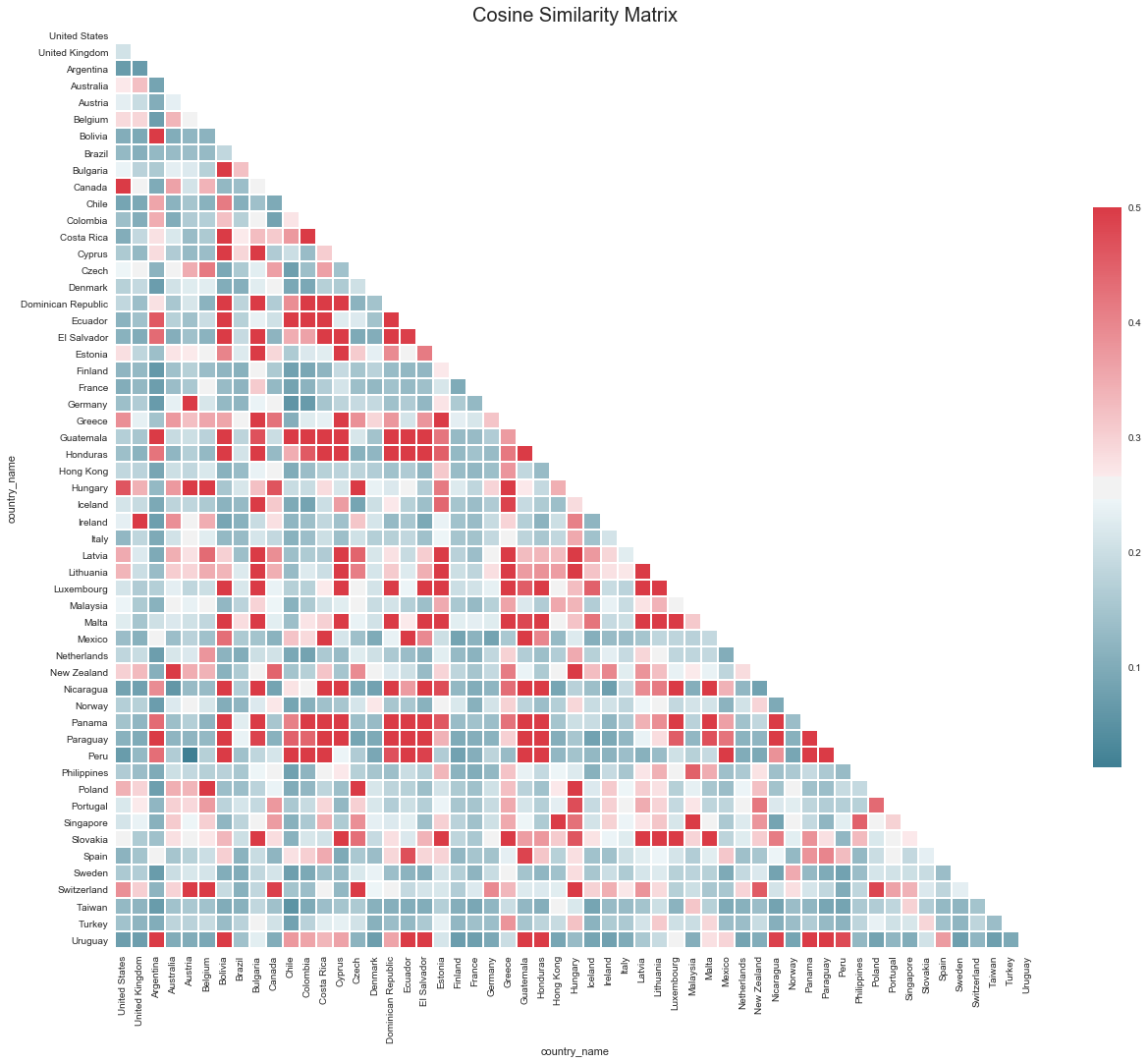I first turn to my favorite country - Colombia - to inspect its similarity with other Latin American countries. The numbers are high for: Peru, Paraguay, Panama, Nicaragua, Gautemala, Ecuador, Dominican Republic and Costa Rica.

Next, I turn to the United Kingdom: it is most similar with Switzerland, New Zealand, Ireland, Hungary, Belgium and Australia.

Finally, we see that Sweden is similar enough to Norway, yet not Finland (who, incidentally, seems to have nothing in common with anyone at all).

Overall, this looks very good to me.

# Visualize with TSNE¶

To further inspect similarities between countries, let's explore the 2-dimensional TSNE space.

In :
tsne = TSNE(perplexity=30, n_components=2, init='pca', n_iter=5000, random_state=12345)

country_embeddings = pd.DataFrame(
data=tsne.fit_transform(country_vectors_df),
index=country_vectors_df.index,
columns=['dim_1', 'dim_2']
)

In :
def plot_tsne_embeddings(country_embeddings):

plt.figure(figsize=(15,15))

for country_name, country_embedding in country_embeddings.iterrows():
dim_1, dim_2 = country_embedding
plt.scatter(dim_1, dim_2)
plt.annotate(country_name, xy=(dim_1, dim_2), xytext=(5, 2), textcoords='offset points', ha='right', va='bottom')
plt.title('Two-Dimensional TSNE Embeddings of Latent Country Vectors', fontsize=16)
plt.xlabel('Dimension 1', fontsize=12)
plt.ylabel('Dimension 2', fontsize=12)
plt.show()

In :
plot_tsne_embeddings(country_embeddings)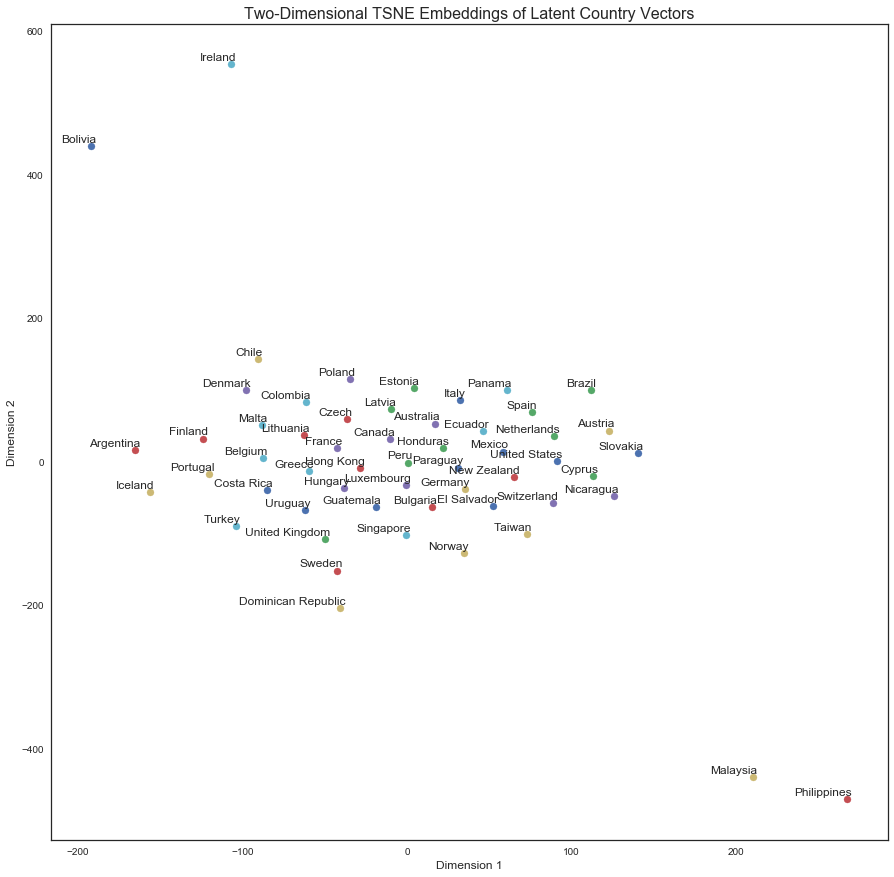This is not particularly useful. Furthermore, and perhaps most importantly, the plot varies immensely when changing the random seed. This is a worthwhile step nonetheless.

# Inspect predicted preferences¶

Crucially, we'll now inspect predicted preferences for select countries and confirm that they make sense. I first normalize each of the country and song vectors, then compute predicted preferences via the dot product.

In [ ]:
SONG_METADATA_QUERY = """
SELECT
songs.title as song_title,
songs.artist as song_artist,
songs.id as song_id
FROM songs
"""

.set_index(['song_title', 'song_artist'])

In :
song_vectors_df_norm = song_vectors_df.apply(lambda vec: vec / np.linalg.norm(vec), axis=1)
country_vectors_df_norm = country_vectors_df.apply(lambda vec: vec / np.linalg.norm(vec), axis=1)

assert song_vectors_df_norm.apply(np.linalg.norm, axis=1).mean() == 1.
assert country_vectors_df_norm.apply(np.linalg.norm, axis=1).mean() == 1.


## United States¶

In :
pd.set_option('display.max_colwidth', 100)

In :
country_vec = country_vectors_df_norm.ix['United States']

Out:
song_title song_artist 0
0 Dab of Ranch - Recorded at Spotify Studios NYC Migos 0.899299
1 Massage In My Room Future 0.899299
2 Father Stretch My Hands Pt. 1 Kanye West 0.899299
3 Keep On Kehlani 0.899299
4 First Day Out Tee Grizzley 0.899299
5 When I Was Broke Future 0.899299
6 Star Of The Show Thomas Rhett 0.899299
7 Rose Golden Kid Cudi 0.899299
8 oui Jeremih 0.899299
9 Everyday We Lit YFN Lucci 0.899299

## Colombia¶

In :
country_vec = country_vectors_df_norm.ix['Colombia']

Out:
song_title song_artist 0
0 Ya No Me Duele Más Silvestre Dangond 0.941204
1 Bajo el agua Manuel Medrano 0.919997
2 Si No Te Quiere Ozuna 0.916971
3 El Ganador Nicky Jam 0.898030
4 Oiga, Mira, Vea Guayacán Orquesta 0.897944
5 Mil Lágrimas Nicky Jam 0.897863
6 Déjame Te Explico Reykon 0.897396
7 Quédate Manuel Medrano 0.896307
8 Afuera del planeta Manuel Medrano 0.895686
9 Materialista Silvestre Dangond 0.895501

## Turkey¶

In :
country_vec = country_vectors_df_norm.ix['Turkey']

Out:
song_title song_artist 0
0 Dudak Edis 0.943574
1 Benim Karanlık Yanım Sezen Aksu 0.943574
2 Affection Cigarettes After Sex 0.943574
3 Ateş Böceği Mithat Can Özer 0.943574
4 Beni Öyle Bilme Ceylan Ertem 0.943574
5 Keyfim Kaçık Acık Can Bonomo 0.943574
6 Seni Unutmaya Ömrüm Yeter mi Ümit Besen 0.943574
7 Vazgeçtim Sezen Aksu 0.943574
8 Yanımda Kal Harun Kolçak 0.943574
9 Stanga Sagi Abitbul 0.943574

## Germany¶

In :
country_vec = country_vectors_df_norm.ix['Germany']

Out:
song_title song_artist 0
0 Skandale Bonez MC 0.908499
1 Ahnma Beginner 0.906591
2 Am schönsten SDP 0.906250
3 Tage wie diese Die Toten Hosen 0.906250
4 Kartell (feat. Azet & Nash) Zuna 0.906250
5 Chill mal dein Leben (feat. Moe Phoenix) Kianush 0.906250
6 Neuanfang Clueso 0.906250
7 Neben der Spur Maxwell 0.906250
8 Hochspannung Maxwell 0.906250
9 Intro Capital Bra 0.906250

## Taiwan¶

In :
country_vec = country_vectors_df_norm.ix['Taiwan']

Out:
song_title song_artist 0
0 如果的事 王藍茵 0.954455
1 小小的 思衛 0.954455
2 Heathens (feat. MUTEMATH) Twenty One Pilots 0.954455
3 其實都沒有 楊宗緯 0.954455
4 對摺 - 國語 任賢齊 0.954455
5 最難的是相遇 Valen Hsu 0.954455
6 안녕 못해 I'm Not Okay (From “MISSING 9”) CHEN 0.954455
7 未接來電 楊乃文 0.954455
8 飛機 - feat. 林俊峰 林俊傑 0.954455
9 All On Me Devin Dawson 0.954455

These look great.

One interesting thing to note is the significance of the length of the vectors. To this point, Schakel and Wilson offer the following:

A word that is consistently used in a similar context will be represented by a longer vector than a word of the same frequency that is used in different contexts.

Not only the direction, but also the length of word vectors carries important information.

Word vector length furnishes, in combination with term frequency, a useful measure of word significance.

In our case, my guess is that length indicates something like popularity - at least for songs. Let's see which songs and countries have the largest L2 norms.

In :
song_vectors_df.apply(np.linalg.norm, axis=1).sort_values(ascending=False).reset_index().head(10)

Out:
song_title song_artist 0
0 Shape of You Ed Sheeran 0.687497
1 Starboy The Weeknd 0.684529
2 I Don’t Wanna Live Forever (Fifty Shades Darker) - From "Fifty Shades Darker (Original Motion Pi... ZAYN 0.682996
3 Despacito (Featuring Daddy Yankee) Luis Fonsi 0.682941
4 Rockabye (feat. Sean Paul & Anne-Marie) Clean Bandit 0.682918
5 Paris The Chainsmokers 0.682878
6 Call On Me - Ryan Riback Extended Remix Starley 0.682799
7 Castle on the Hill Ed Sheeran 0.682442
8 I Feel It Coming The Weeknd 0.682225
9 Closer The Chainsmokers 0.682175
In :
country_vectors_df.apply(np.linalg.norm, axis=1).sort_values(ascending=False).reset_index().head(10)

Out:
country_name 0
0 Taiwan 4.842656
1 Sweden 4.602653
2 Finland 4.415459
3 France 4.399233
4 United Kingdom 4.374870
5 Netherlands 4.330190
6 Denmark 4.232457
7 Italy 4.218088
8 Norway 4.084380
9 Germany 4.076692

To reference Taiwan and Colombia, let's inspect predictions using unnormalized vectors.

In :
country_vec = country_vectors_df.ix['Taiwan']

Out:
song_title song_artist 0
0 Bad Things - With Camila Cabello Machine Gun Kelly 0.958864
1 Just Hold On Steve Aoki 0.958692
2 Shape of You Ed Sheeran 0.958325
3 Side To Side Ariana Grande 0.957497
4 Closer The Chainsmokers 0.957290
5 Starboy The Weeknd 0.957090
6 Paris The Chainsmokers 0.957001
7 I Don’t Wanna Live Forever (Fifty Shades Darker) - From "Fifty Shades Darker (Original Motion Pi... ZAYN 0.956933
8 Something Just Like This The Chainsmokers 0.956803
9 The Greatest Sia 0.956746
In :
country_vec = country_vectors_df.ix['Colombia']

Out:
song_title song_artist 0
0 I Got You Bebe Rexha 1.019838
1 Party Monster The Weeknd 0.993647
3 I Would Like Zara Larsson 0.987099
4 Scars To Your Beautiful Alessia Cara 0.987036
5 Weak AJR 0.986642
6 Too Good Drake 0.982179
7 Nancy Mulligan Ed Sheeran 0.979373
8 Supermarket Flowers Ed Sheeran 0.978706
9 Hearts Don't Break Around Here Ed Sheeran 0.978494

Respectively, there is not a song with Chinese nor Spanish letters in each set of recommendations. Additionally, it seems like the world is fond of Ed Sheeran. It is clear that the global popularity of songs dominates our recommendations when computing the dot product with unnormalized vectors.

When we do normalize, our predicted preferences can be defined thus:

$$\hat{x}_u = \frac{x_u}{\|x_u\|} \quad \hat{y}_i = \frac{y_i}{\|y_i\|}\\ \hat{p}_{u, i} = \hat{x}_u \cdot \hat{y}_i$$

One idea might be to scale the song vectors by their respective lengths, leaving our predicted preferences as:

\begin{align*} \hat{p}_{u, i} &= \hat{x}_u \cdot \big(\hat{y}_i \cdot c\|y_i\|\big)\\ &= \hat{x}_u \cdot \Bigg(\hat{y}_i \cdot c\frac{y_i}{\hat{y_i}}\Bigg)\\ &= \hat{x}_u \cdot c y_i \end{align*}

Let's briefly see what this looks like.

In :
C = .2

country_vec = country_vectors_df_norm.ix['Taiwan']

Out:
song_title song_artist 0
0 Bad Things - With Camila Cabello Machine Gun Kelly 0.039601
1 Just Hold On Steve Aoki 0.039594
2 Shape of You Ed Sheeran 0.039578
3 Side To Side Ariana Grande 0.039544
4 Closer The Chainsmokers 0.039536
5 Starboy The Weeknd 0.039528
6 Paris The Chainsmokers 0.039524
7 I Don’t Wanna Live Forever (Fifty Shades Darker) - From "Fifty Shades Darker (Original Motion Pi... ZAYN 0.039521
8 Something Just Like This The Chainsmokers 0.039516
9 The Greatest Sia 0.039513

About the same. Nevertheless, the length of our vectors can likely offer some value when balancing local recommendations with globally popular songs. I leave this to the reader to explore further for now.

# Approximating IMF with neural networks¶

Again, IMF gives a function $f: u, i \rightarrow \hat{p}_{u, i}$ and the following objective function to minimize:

$$\underset{x_{*}, y_{*}}{\arg\min}\sum\limits_{u, i}c_{u, i}\big(p_{u, i} - f(u, i)\big)^2 + \lambda\bigg(\sum\limits_u\|x_u\|^2 + \sum\limits_i\|y_u\|^2\bigg)$$

Let's try to do this with neural networks. I'll use the $\alpha$ and $\lambda$ parameters found above, and keep the same dimensionality, $F = 30$, for our latent vectors. This number was chosen in separate experimentation not shown here.

In :
from itertools import product

from keras import backend as K
from keras.layers import Input, Embedding, Flatten, Lambda, Dense, BatchNormalization, Dropout, LSTM
from keras.layers.merge import add, dot, concatenate
from keras.models import Model
from keras.preprocessing.text import Tokenizer
from keras.regularizers import l2
from keras.utils import plot_model

In :
songs_rated_by_country = (ratings_matrix.R_ui > 0).sum(axis=1)

plt.hist(songs_rated_by_country)
plt.title('Distribution of # of Unique Songs Streamed per Country', fontsize=15)
plt.xlabel('Unique Songs Streamed', fontsize=12)
_ = plt.ylabel('# of Countries', fontsize=12)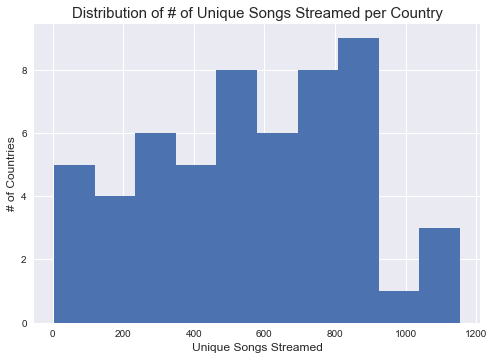In :
print('The 15th percentile of songs rated by country is {}.'.format(
np.percentile(songs_rated_by_country, 15)
))

The 15th percentile of songs rated by country is 218.20000000000002.


Let's set our eligibility cutoff at 200 songs and create training and validation matrices.

In :
more_than_200_ratings_mask = songs_rated_by_country > 20

training_matrix, validation_matrix = split_ratings_matrix_into_training_and_validation(
ratings_matrix=ratings_matrix,
)


## Arrange our data¶

To interact with Keras, we'll need to change our data from wide format to long. Additionally, so as to create our embeddings, we'll need to give each country and song a unique, contiguous index. Finally, I'll do the same with the song artist, as well as tokenize the song title, for later use.

In :
song_metadata_df = song_metadata_df.ix[ratings_matrix.R_ui.columns]

Out:
song_title song_artist
song_id
5552 El Perdón Nicky Jam
5553 Lean On (feat. MØ & DJ Snake) Major Lazer
5555 Love Me Like You Do - From "Fifty Shades Of Grey" Ellie Goulding
5556 Uptown Funk Mark Ronson
5557 Loquita Marama
In :
country_id_to_index = {c_id: index for index, c_id in enumerate(ratings_matrix.R_ui.index)}
song_id_to_index = {s_id: index for index, s_id in enumerate(ratings_matrix.R_ui.columns)}
song_artist_to_index = {artist: index for index, artist in enumerate(song_metadata_df['song_artist'].unique())}


Out:
song_title song_artist song_index song_artist_index
song_id
33068 Affection Cigarettes After Sex 8658 3699
33069 Istemem Soz Sevmeni Ferman Akgül 8659 3106
33070 Skammekroken 2017 TIX 8660 1677
33071 Wiosna Organek 8661 3716
33072 Fuckt. Strapo 8662 3717

### Tokenize song title¶

In :
NUM_WORDS = 5000

tokenizer = Tokenizer(num_words=NUM_WORDS, lower=True, split=" ")


### Convert ratings to long format¶

In :
input_tuples = list( product(ratings_matrix.R_ui.index, ratings_matrix.R_ui.columns) )
country_ids, song_ids = zip(*input_tuples)

ratings_df = pd.DataFrame({
'country_id': country_ids,
'song_id': song_ids,
'train_rating': training_matrix.R_ui.values.flatten(),
'validation_rating': validation_matrix.R_ui.values.flatten(),
})

ratings_df['country_index'] = ratings_df['country_id'].map(country_id_to_index.get)


## Define network parameters¶

In :
n_countries = ratings_df['country_index'].nunique()
n_songs = ratings_df['song_index'].nunique()
n_artists = ratings_df['song_artist_index'].nunique()

alpha = best_params.alpha
lmbda = best_params.lmbda
F = 30
LEARNING_RATE = .001


## Define a loss function¶

This is simply the IMF objective we'd like to minimize.

In :
def implicit_cf_loss(y_true, y_pred, alpha=alpha):
P = y_true > 0
C = 1 + alpha*y_true
return K.mean( C*(P - y_pred)**2 )


## Define functions to generate, evaluate and visualize predictions¶

In :
def generate_predictions(model, inputs, ratings_df=ratings_df):
predictions = model.predict(x=inputs)
return pd.DataFrame({
'prediction': predictions.flatten(),
'country_id': ratings_df['country_id'],
'song_id': ratings_df['song_id']
}).pivot('country_id', 'song_id', 'prediction')

In :
def evaluate_predictions(predictions, eligibility_mask=more_than_200_ratings_mask,
training_matrix=training_matrix, validation_matrix=validation_matrix):
evaluator = ExpectedPercentileRankingsEvaluator(
training_matrix=training_matrix,
validation_matrix=validation_matrix,
predicted_preferences=predictions
)
evaluator.run()
print('Train: {}'.format(evaluator.mean_expected_percentile_rankings_train))
print('Validation: {}'.format(evaluator.mean_expected_percentile_rankings_validation))

In :
def visualize_predictions(predictions):
plt.figure(figsize=(10, 6))

plt.hist(predictions.values.flatten(), bins=25)
plt.title('Predicted Preferences Histogram', fontsize=15)
plt.xlabel('Predicted Prefence', fontsize=12)
_ = plt.ylabel('Count', fontsize=12)


# Network #1¶

A trivially "Siamese" network which first embeds each country and song index into $\mathbb{R}^f$ in parallel then computes a dot-product of the embeddings. This is roughly equivalent to what is being done by IMF.

In :
country_input = Input(shape=(1,), dtype='int64', name='country')
song_input = Input(shape=(1,), dtype='int64', name='song')

country_embedding = Embedding(input_dim=n_countries, output_dim=F, embeddings_regularizer=l2(lmbda))(country_input)
song_embedding = Embedding(input_dim=n_songs, output_dim=F, embeddings_regularizer=l2(lmbda))(song_input)

predicted_preference = dot(inputs=[country_embedding, song_embedding], axes=2)
predicted_preference = Flatten()(predicted_preference)

In :
model = Model(inputs=[country_input, song_input], outputs=predicted_preference)

plot_model(model, to_file='figures/network_1.png')In :
inputs = [ratings_df['country_index'], ratings_df['song_index']]

network_1 = model.fit(
x=inputs,
y=ratings_df['train_rating'],
batch_size=1024,
epochs=5,
validation_data=(inputs, ratings_df['validation_rating'])
)

Train on 476465 samples, validate on 476465 samples
Epoch 1/5
476465/476465 [==============================] - 2s - loss: 64.9000 - val_loss: 0.0185
Epoch 2/5
476465/476465 [==============================] - 8s - loss: 0.0744 - val_loss: 0.0185
Epoch 3/5
476465/476465 [==============================] - 3s - loss: 0.0744 - val_loss: 0.0185
Epoch 4/5
476465/476465 [==============================] - 10s - loss: 0.0745 - val_loss: 0.0185
Epoch 5/5
476465/476465 [==============================] - 8s - loss: 0.0745 - val_loss: 0.0186

In :
predictions = generate_predictions(model=model, inputs=inputs)
evaluate_predictions(predictions)

Train: 0.5053388101677021
Validation: 0.49071882278215817


For reference, let's recall the results we computed when validation our model with best_params.

In :
grid_search_results[best_params]

Out:
{'train': 0.04479720392806505, 'validation': 0.18543168148626762}

Additionally, a random model should return an expected percentile ranking of ~50%, as noted in Hu, Koren and Volinksy. So, this is really bad. Let's visualize the distribution of predictions before moving on to another model.

In :
visualize_predictions(predictions)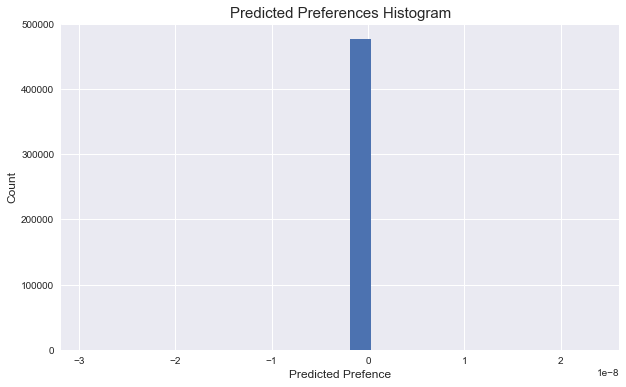# Network #2¶

Same as previous, but with a bias embedding for each set, in $\mathbb{R}$, added to the dot-product.

In :
country_bias = Embedding(input_dim=n_countries, output_dim=1, name='country_bias', input_length=1)(country_input)
song_bias = Embedding(input_dim=n_songs, output_dim=1, name='song_bias', input_length=1)(song_input)

predicted_preference = Flatten()(predicted_preference)

In :
model = Model(inputs=[country_input, song_input], outputs=predicted_preference)

plot_model(model, to_file='figures/network_2.png')In :
inputs = [ratings_df['country_index'], ratings_df['song_index']]

network_2 = model.fit(
x=inputs,
y=ratings_df['train_rating'],
batch_size=1024,
epochs=5,
validation_data=(inputs, ratings_df['validation_rating'])
)

Train on 476465 samples, validate on 476465 samples
Epoch 1/5
476465/476465 [==============================] - 2s - loss: 0.0734 - val_loss: 0.0204
Epoch 2/5
476465/476465 [==============================] - 2s - loss: 0.0563 - val_loss: 0.0216
Epoch 3/5
476465/476465 [==============================] - 2s - loss: 0.0534 - val_loss: 0.0236
Epoch 4/5
476465/476465 [==============================] - 2s - loss: 0.0515 - val_loss: 0.0252
Epoch 5/5
476465/476465 [==============================] - 2s - loss: 0.0502 - val_loss: 0.0269

In :
predictions = generate_predictions(model=model, inputs=inputs)
evaluate_predictions(predictions)

Train: 0.10900494586591782
Validation: 0.1906236846450195


This looks a lot better. Now let's visualize our ground-truth and predictions side-by-side.

In :
_ = pd.DataFrame({
'predictions': predictions.values.flatten(),
'ratings': ratings_df['train_rating'].values.flatten()
}).hist(figsize=(16, 6))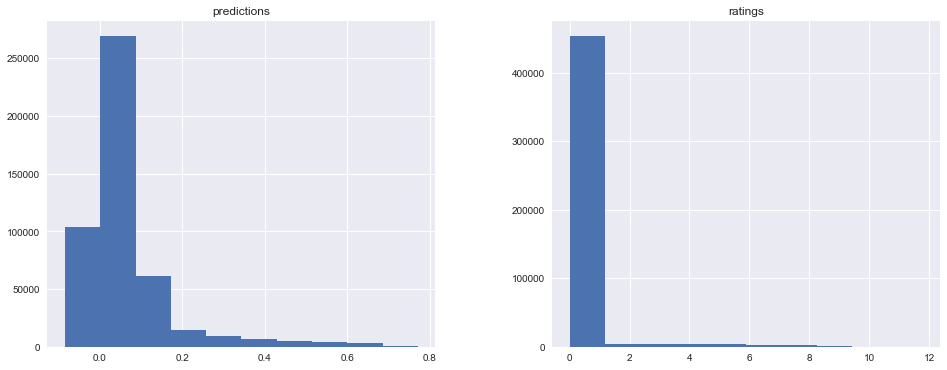This model approximates the training distribution much better than the others. Key to this task is delineating between items with 0 ratings, and those with high ratings. Remember, we first down-scaled our ratings with the following transformation to shrink the domain:

$$\tilde{r}_{u, i} = \log{\bigg(\frac{1 + r_{u, i}}{\epsilon}\bigg)}$$

Furthermore, the model scores worse on the training distribution, yet similarly on the validation set. The bias term was clearly helpful.

Let's try a similar model, but concatenate the country and song embeddings, then stack fully connected layers, then compute the final linear combination as before.

# Network #3¶

Same as #1, but concatenate the latent vectors instead. Then, stack 3 fully-connected layers with ReLU activations, batch normalization after each, and dropout after the first. Finally, add a 1-unit dense layer on the end, and add bias embeddings to the result. (NB: I wanted to add the bias embeddings to the respective $\mathbb{R}^f$ embeddings at the outset, but couldn't figure out how to do this in Keras.)

In :
country_input = Input(shape=(1,), dtype='int64', name='country')
song_input = Input(shape=(1,), dtype='int64', name='song')

country_embedding = Embedding(input_dim=n_countries, output_dim=F, embeddings_regularizer=l2(lmbda))(country_input)
song_embedding = Embedding(input_dim=n_songs, output_dim=F, embeddings_regularizer=l2(lmbda))(song_input)

country_bias = Embedding(input_dim=n_countries, output_dim=1, name='country_bias', input_length=1)(country_input)
song_bias = Embedding(input_dim=n_songs, output_dim=1, name='song_bias', input_length=1)(song_input)

concatenation = concatenate([country_embedding, song_embedding])

dense_layer = Dense(activation='relu', units=10)(concatenation)
dropout = Dropout(.5)(dense_layer)
batch_norm = BatchNormalization()(dropout)
dense_layer = Dense(activation='relu', units=10)(batch_norm)
batch_norm = BatchNormalization()(dense_layer)
dense_layer = Dense(activation='relu', units=10)(batch_norm)
batch_norm = BatchNormalization()(dense_layer)

predicted_preference = Dense(units=1, name='predicted_preference')(batch_norm)
predicted_preference = Flatten()(predicted_preference)

predicted_preference = Flatten()(predicted_preference)

In :
model = Model(inputs=[country_input, song_input], outputs=predicted_preference)

plot_model(model, to_file='figures/network_3.png')In :
inputs = [ratings_df['country_index'], ratings_df['song_index']]

network_3 = model.fit(
x=inputs,
y=ratings_df['train_rating'],
batch_size=1024,
epochs=5,
validation_data=(inputs, ratings_df['validation_rating'])
)

Train on 476465 samples, validate on 476465 samples
Epoch 1/5
476465/476465 [==============================] - 5s - loss: 0.0702 - val_loss: 0.0830
Epoch 2/5
476465/476465 [==============================] - 5s - loss: 0.0472 - val_loss: 0.0601
Epoch 3/5
476465/476465 [==============================] - 5s - loss: 0.0477 - val_loss: 0.0667
Epoch 4/5
476465/476465 [==============================] - 5s - loss: 0.0483 - val_loss: 0.0508
Epoch 5/5
476465/476465 [==============================] - 5s - loss: 0.0491 - val_loss: 0.0513

In :
predictions = generate_predictions(model=model, inputs=inputs)
evaluate_predictions(predictions)

Train: 0.11102242413459021
Validation: 0.1899202531334214


These results are roughly identical to the previous. Choosing between the models, we'd certainly go with the simpler of the two.

Now, let's try some architectures that benefit from other data sources altogether: the song title, and the song artist.

# Network #4¶

Same as #2, except feed in the song title text as well. This text is first tokenized, then padded to a maximum sequence length, then embedded into a fixed-length vector by an LSTM, then reduced to a single value by a dense layer with a ReLU activation. Finally, this scalar is concatenated to the scalar output that #2 would produce, and the result is fed into a final dense layer with a linear activation - i.e. a linear combination of the two.

In :
MAX_SEQUENCE_LENGTH = ratings_df['title_sequence'].map(len).max()


In :
country_input = Input(shape=(1,), dtype='int64', name='country')
song_input = Input(shape=(1,), dtype='int64', name='song')
title_input = Input(shape=(MAX_SEQUENCE_LENGTH,), dtype='int32', name='song_title_sequence')

country_embedding = Embedding(input_dim=n_countries, output_dim=F, embeddings_regularizer=l2(lmbda))(country_input)
song_embedding = Embedding(input_dim=n_songs, output_dim=F, embeddings_regularizer=l2(lmbda))(song_input)
title_embedding = Embedding(output_dim=F, input_dim=NUM_WORDS, input_length=MAX_SEQUENCE_LENGTH)(title_input)

country_bias = Embedding(input_dim=n_countries, output_dim=1, name='country_bias', input_length=1)(country_input)
song_bias = Embedding(input_dim=n_songs, output_dim=1, name='song_bias', input_length=1)(song_input)

predicted_preference = dot(inputs=[country_embedding, song_embedding], axes=2)
predicted_preference = Flatten()(predicted_preference)

title_lstm = LSTM(F)(title_embedding)
dense_title_lstm = Dense(units=1, activation='relu')(title_lstm)

predicted_preference_merge = concatenate(inputs=[predicted_preference, dense_title_lstm])
final_output = Dense(activation='linear', units=1)(predicted_preference_merge)

In :
model = Model(inputs=[country_input, song_input, title_input], outputs=final_output)

plot_model(model, to_file='figures/network_4.png')In :
inputs = [ratings_df['country_index'], ratings_df['song_index'], padded_title_sequences]

network_4 = model.fit(
x=inputs,
y=ratings_df['train_rating'],
batch_size=1024,
epochs=5,
validation_data=(inputs, ratings_df['validation_rating'])
)

Train on 476465 samples, validate on 476465 samples
Epoch 1/5
476465/476465 [==============================] - 124s - loss: 65.4419 - val_loss: 0.0215
Epoch 2/5
476465/476465 [==============================] - 126s - loss: 0.0506 - val_loss: 0.0256
Epoch 3/5
476465/476465 [==============================] - 120s - loss: 0.0476 - val_loss: 0.0282
Epoch 4/5
476465/476465 [==============================] - 119s - loss: 0.0472 - val_loss: 0.0288
Epoch 5/5
476465/476465 [==============================] - 119s - loss: 0.0471 - val_loss: 0.0291

In :
predictions = generate_predictions(model=model, inputs=inputs)
evaluate_predictions(predictions)

Train: 0.11471700578472085
Validation: 0.18885209182480478


We seem to get a pinch of lift from the song title, though we're still underfitting our training set. Perhaps we could make our model bigger, or run it for more epochs.

Let's plot our ground-truth vs. prediction distributions side-by-side just to make sure we're still on the right track.

In :
_ = pd.DataFrame({
'predictions': predictions.values.flatten(),
'ratings': ratings_df['train_rating'].values.flatten()
}).hist(figsize=(16, 6))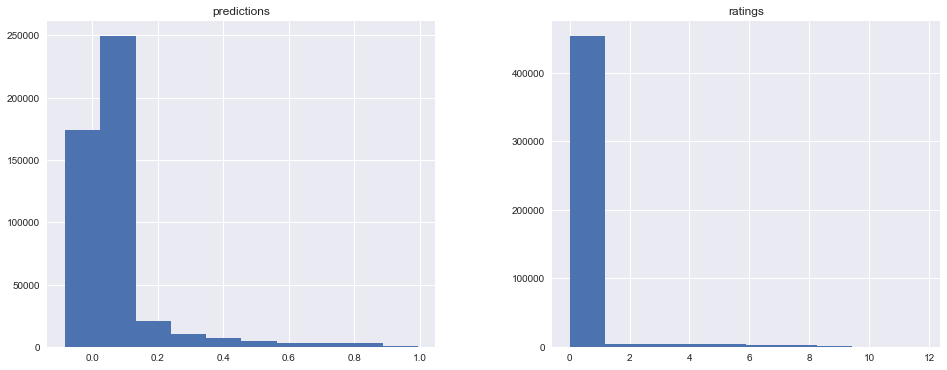# Network #5¶

Same as #4, except feed in the song artist index as well. This index is first embedded into a vector, then reduced to a scalar by a dense layer with a ReLU activation. Finally, this scalar is concatenated with the two scalars produced in the second-to-last layer of #4, then fed into a final dense layer with a linear activation. Like the previous, this is a linear combination of the three inputs.

In :
artist_input = Input(shape=(1,), dtype='int64', name='artist')
artist_embedding = Embedding(input_dim=n_artists, output_dim=F, embeddings_regularizer=l2(lmbda))(artist_input)
dense_artist_embedding = Dense(units=1, activation='relu')(artist_embedding)
dense_artist_embedding = Flatten()(dense_artist_embedding)

predicted_preference_merge = concatenate(inputs=[predicted_preference, dense_title_lstm, dense_artist_embedding])
final_output = Dense(activation='linear', units=1)(predicted_preference_merge)

In :
model = Model(inputs=[country_input, song_input, title_input, artist_input], outputs=final_output)

plot_model(model, to_file='figures/network_5.png')In :
inputs = [
ratings_df['country_index'],
ratings_df['song_index'],
ratings_df['song_artist_index']
]

model.fit(
x=inputs,
y=ratings_df['train_rating'],
batch_size=1024,
epochs=5,
validation_data=(inputs, ratings_df['validation_rating'])
)

Train on 476465 samples, validate on 476465 samples
Epoch 1/5
476465/476465 [==============================] - 122s - loss: 28.0315 - val_loss: 0.0224
Epoch 2/5
476465/476465 [==============================] - 129s - loss: 0.0484 - val_loss: 0.0269
Epoch 3/5
476465/476465 [==============================] - 121s - loss: 0.0473 - val_loss: 0.0310
Epoch 4/5
476465/476465 [==============================] - 134s - loss: 0.0489 - val_loss: 0.0333
Epoch 5/5
476465/476465 [==============================] - 131s - loss: 0.0485 - val_loss: 0.0300

Out:
<keras.callbacks.History at 0x12ee912b0>
In :
predictions = generate_predictions(model=model, inputs=inputs)
evaluate_predictions(predictions)

Train: 0.11092262775206822
Validation: 0.19037930919362725


This seems a bit worse than the last, though similar all the same. I'm a bit surprised we didn't get more lift from artists. Nevertheless, we're still underfitting. Bigger model, more epochs, etc.

# What went wrong?¶

Our best neural network in terms of performance and complexity is network #2. Let's refit this model and inspect a few predictions.

In :
country_input = Input(shape=(1,), dtype='int64', name='country')
song_input = Input(shape=(1,), dtype='int64', name='song')

country_embedding = Embedding(input_dim=n_countries, output_dim=F, embeddings_regularizer=l2(lmbda))(country_input)
song_embedding = Embedding(input_dim=n_songs, output_dim=F, embeddings_regularizer=l2(lmbda))(song_input)

country_bias = Embedding(input_dim=n_countries, output_dim=1, name='country_bias', input_length=1)(country_input)
song_bias = Embedding(input_dim=n_songs, output_dim=1, name='song_bias', input_length=1)(song_input)

predicted_preference = dot(inputs=[country_embedding, song_embedding], axes=2)
predicted_preference = Flatten()(predicted_preference)

predicted_preference = Flatten()(predicted_preference)

In :
model = Model(inputs=[country_input, song_input], outputs=predicted_preference)

In :
inputs = [ratings_df['country_index'], ratings_df['song_index']]

network_2 = model.fit(
x=inputs,
y=ratings_df['train_rating'],
batch_size=1024,
epochs=5,
validation_data=(inputs, ratings_df['validation_rating'])
)

Train on 476465 samples, validate on 476465 samples
Epoch 1/5
476465/476465 [==============================] - 3s - loss: 65.2042 - val_loss: 0.0205
Epoch 2/5
476465/476465 [==============================] - 8s - loss: 0.0559 - val_loss: 0.0212
Epoch 3/5
476465/476465 [==============================] - 3s - loss: 0.0519 - val_loss: 0.0225
Epoch 4/5
476465/476465 [==============================] - 2s - loss: 0.0496 - val_loss: 0.0235
Epoch 5/5
476465/476465 [==============================] - 2s - loss: 0.0482 - val_loss: 0.0248

In :
predictions = generate_predictions(model=model, inputs=inputs)
evaluate_predictions(predictions)

Train: 0.10905118689288228
Validation: 0.19050085125816704

In :
predictions_unstack = predictions.unstack()

predictions_unstack = pd.DataFrame({
'country': unstack.index.get_level_values('country_id').map(country_id_to_name.get),
'rating': predictions_unstack.values
})


## Colombia¶

In :
predictions_unstack[predictions_unstack['country'] == 'Colombia'].sort_values(by='rating', ascending=False).head(10)

Out:
country rating song_artist song_title
song_id
30519 Colombia 0.715299 Katy Perry Chained To The Rhythm
31060 Colombia 0.704788 Ed Sheeran Perfect
30137 Colombia 0.683443 Ed Sheeran Castle on the Hill
31043 Colombia 0.681572 Zedd Stay (with Alessia Cara)
31041 Colombia 0.680419 The Chainsmokers Something Just Like This
18894 Colombia 0.674962 Calvin Harris My Way
30487 Colombia 0.674799 Major Lazer Run Up (feat. PARTYNEXTDOOR & Nicki Minaj)
20131 Colombia 0.672363 The Weeknd I Feel It Coming
20222 Colombia 0.671484 Bruno Mars That's What I Like
31045 Colombia 0.669374 Clean Bandit Symphony (feat. Zara Larsson)

## United States¶

In :
predictions_unstack[predictions_unstack['country'] == 'United States'].sort_values(by='rating', ascending=False).head(10)

Out:
country rating song_artist song_title
song_id
30519 United States 0.752865 Katy Perry Chained To The Rhythm
31060 United States 0.742354 Ed Sheeran Perfect
30137 United States 0.721009 Ed Sheeran Castle on the Hill
31043 United States 0.719138 Zedd Stay (with Alessia Cara)
31041 United States 0.717985 The Chainsmokers Something Just Like This
18894 United States 0.712528 Calvin Harris My Way
30487 United States 0.712365 Major Lazer Run Up (feat. PARTYNEXTDOOR & Nicki Minaj)
20131 United States 0.709929 The Weeknd I Feel It Coming
20222 United States 0.709051 Bruno Mars That's What I Like
31045 United States 0.706940 Clean Bandit Symphony (feat. Zara Larsson)

## Turkey¶

In :
predictions_unstack[predictions_unstack['country'] == 'Turkey'].sort_values(by='rating', ascending=False).head(10)

Out:
country rating song_artist song_title
song_id
30519 Turkey 0.728861 Katy Perry Chained To The Rhythm
31060 Turkey 0.718350 Ed Sheeran Perfect
30137 Turkey 0.697005 Ed Sheeran Castle on the Hill
31043 Turkey 0.695134 Zedd Stay (with Alessia Cara)
31041 Turkey 0.693981 The Chainsmokers Something Just Like This
18894 Turkey 0.688524 Calvin Harris My Way
30487 Turkey 0.688361 Major Lazer Run Up (feat. PARTYNEXTDOOR & Nicki Minaj)
20131 Turkey 0.685925 The Weeknd I Feel It Coming
20222 Turkey 0.685046 Bruno Mars That's What I Like
31045 Turkey 0.682936 Clean Bandit Symphony (feat. Zara Larsson)

## Taiwan¶

In :
predictions_unstack[predictions_unstack['country'] == 'Taiwan'].sort_values(by='rating', ascending=False).head(10)

Out:
country rating song_artist song_title
song_id
30519 Taiwan 0.789601 Katy Perry Chained To The Rhythm
31060 Taiwan 0.779090 Ed Sheeran Perfect
30137 Taiwan 0.757745 Ed Sheeran Castle on the Hill
31043 Taiwan 0.755874 Zedd Stay (with Alessia Cara)
31041 Taiwan 0.754721 The Chainsmokers Something Just Like This
18894 Taiwan 0.749264 Calvin Harris My Way
30487 Taiwan 0.749101 Major Lazer Run Up (feat. PARTYNEXTDOOR & Nicki Minaj)
20131 Taiwan 0.746664 The Weeknd I Feel It Coming
20222 Taiwan 0.745786 Bruno Mars That's What I Like
31045 Taiwan 0.743676 Clean Bandit Symphony (feat. Zara Larsson)

As is abundantly clear, the model is simply converging to a popularity baseline. I tried extracting the vectors outright, normalizing as before then computing dot products, but the results made little sense.

# Future work¶

At this stage, Implicit Matrix Factorization is the clear winner. The algorithm is popular for several good reasons - it's simple, relatively scalable, intuitive (especially given the significance of the interplay between $C$ and $P$, which I did not expressedly discuss) and effective. Herein, I did not produce a neural network approximating this algorithm with superior results.

My intuition tells me I'm missing something simple; why shouldn't a non-linear function approximator be able to approximate $f(u, i)$ better than a linear matrix decomposition? At this stage, I'm not sure. My best guess is that we should seek to optimize a different loss function. Here, we've mirrored that of IMF; why not optimize a ranking loss function instead, since that's what's ultimately important? The IMF objective makes the actual optimization process easy for ratings matrices with billions of items; in our case, with far fewer items, we're simply using stochastic gradient descent. To this effect, I did experiment with some composite loss functions; on one side, implicit_mf_loss, and on the other, a cutoff_loss, which seeks to enforce that songs which received a rating $\tilde{r}_{u, i}$ above a certain cutoff should be indeed predicted at above that cutoff. It's a crude, binary absolute error. This was unsuccessful.

Finally, if there is in fact some value in using neural networks for this type of problem, I'm extremely intrigued by the ease and intuitiveness with which we can incorporate multiple inputs into our function. Encoding our song title with an LSTM, and training that LSTM end-to-end with our main minimization objective, is one example of this. Furthermore, Keras makes the construction of these networks straightforward.

Thanks for reading this work. Your feedback is welcome, and I do hope this serves as a point of inspiration, trial and error should this question interest you.